# 具有全状态时变约束非线性不确定电机系统的自适应容错控制Adaptive Fault-Tolerant Control for Nonlinear Uncertain Electromechanical Systems with Full-State Time-Varying Constraints

DOI: 10.12677/DSC.2019.84025, PDF, HTML, XML, 下载: 359  浏览: 503  国家自然科学基金支持

Abstract: This paper proposes an adaptive fault-tolerant control method for nonlinear electromechanical system with full-state time varying constraints. In order to ensure the control performance of the system, a fault tolerant compensation controller is constructed to eliminate the influence of the actuator loss of effectiveness and bias fault. The time-varying barrier Lyapunov functions are in-troduced to ensure that all the system state does not exceed the specified time-varying constraint range; especially in the case of actuator failure, the full-state constraints are not violated. Neural network as the approximator is employed to approximate unknown function in the processing system. Based on the Lyapunov analysis, it is proved that all the signals in the closed-loop system are bounded and the good tracking performance of the system is achieved. The simulation results further illustrate the effectiveness of the proposed control strategy.

1. 引言

2. 问题描述及预备知识

$\left\{\begin{array}{l}\left(\frac{J}{{K}_{\tau }}+\frac{{M}_{0}{L}_{0}^{2}}{3{K}_{\tau }}+\frac{2{M}_{0}{R}_{0}^{2}}{5{K}_{\tau }}\right)\stackrel{¨}{q}+\left(\frac{{B}_{0}}{{K}_{\tau }}\right)\stackrel{˙}{q}+\left(\frac{m{L}_{0}G}{2{K}_{\tau }}+\frac{{M}_{0}{L}_{0}G}{{K}_{\tau }}\right)\mathrm{sin}\left(q\right)=I\\ L\stackrel{˙}{I}=\tau -RI-{K}_{B}\stackrel{˙}{q}\end{array}$ (1)

$\left\{\begin{array}{l}{\stackrel{˙}{x}}_{1}={x}_{2}\\ {\stackrel{˙}{x}}_{2}=\frac{1}{M}{x}_{3}-\frac{N}{M}\mathrm{sin}\left({x}_{1}\right)-\frac{B}{M}{x}_{2}\\ {\stackrel{˙}{x}}_{3}=\frac{1}{L}\tau -\frac{{K}_{B}}{L}{x}_{2}-\frac{R}{L}{x}_{3}\\ y={x}_{1}\end{array}$ (2)

$|{x}_{i}|\le {\psi }_{i}\left(t\right),i=1,2,3$ (3)

1) 闭环系统中所有信号有界；

2) 跟踪误差和估计误差尽可能小；

3) 系统状态不超出指定的约束范围。

$\tau =lu+\zeta ,\forall t\ge T$ (4)

$l=1$ ，执行器没有任何失效。例如 $l=0.8$ ，执行器效率失效20%。

3. 自适应神经网络控制器设计及稳定性分析

${\stackrel{˙}{e}}_{1}={\stackrel{˙}{x}}_{1}-{\stackrel{˙}{y}}_{d}={e}_{2}+{\chi }_{1}-{\stackrel{˙}{y}}_{r}$ (5)

${V}_{1}=\frac{{\lambda }_{1}^{2}}{\text{π}}\mathrm{tan}\left(\frac{\text{π}{e}_{1}^{2}}{2{\lambda }_{1}^{2}}\right)$ (6)

${\stackrel{˙}{V}}_{1}=\frac{2{\lambda }_{1}{\stackrel{˙}{\lambda }}_{1}}{\text{π}}tan\left(\frac{\text{π}{e}_{1}^{2}}{2{\lambda }_{1}^{2}}\right)+{\mathrm{sec}}^{2}\left(\frac{\text{π}{e}_{1}^{2}}{2{\lambda }_{1}^{2}}\right){e}_{1}{e}_{2}-\frac{{e}_{1}^{2}{\stackrel{˙}{\lambda }}_{1}}{{\lambda }_{1}}{\mathrm{sec}}^{2}\left(\frac{\text{π}{e}_{1}^{2}}{2{\lambda }_{1}^{2}}\right)+{\mathrm{sec}}^{2}\left(\frac{\text{π}{e}_{1}^{2}}{2{\lambda }_{1}^{2}}\right){e}_{1}\left({\chi }_{1}-{\stackrel{˙}{y}}_{r}\right)$ (7)

${\chi }_{1}=-\left({\rho }_{1}+2{\stackrel{¯}{\lambda }}_{1}\right)\frac{{\lambda }_{1}^{2}}{2\text{π}{e}_{1}}\mathrm{sin}\left(\frac{\text{π}{e}_{1}^{2}}{{\lambda }_{1}^{2}}\right)-{e}_{1}{\stackrel{¯}{\lambda }}_{1}+{\stackrel{˙}{y}}_{r}$ (8)

$\underset{e\to 0}{\mathrm{lim}}\frac{{\lambda }_{1}^{2}}{2\text{π}{e}_{1}}\mathrm{sin}\left(\frac{\text{π}{e}_{1}^{2}}{{\lambda }_{1}^{2}}\right)=0$

${\stackrel{˙}{V}}_{1}=-\frac{{\rho }_{1}{\lambda }_{1}^{2}}{\text{π}}\mathrm{tan}\left(\frac{\text{π}{e}_{1}^{2}}{2{\lambda }_{1}^{2}}\right)+{\mathrm{sec}}^{2}\left(\frac{\text{π}{e}_{1}^{2}}{2{\lambda }_{1}^{2}}\right){e}_{1}{e}_{2}$ (9)

${\stackrel{˙}{e}}_{2}={\stackrel{˙}{x}}_{2}-{\stackrel{˙}{\chi }}_{1}=\frac{1}{M}{x}_{3}-\frac{N}{M}\mathrm{sin}\left({x}_{1}\right)-\frac{B}{M}{x}_{2}-{\stackrel{˙}{\chi }}_{1}$ (10)

${V}_{2}=\frac{{\lambda }_{2}^{2}}{\text{π}}\mathrm{tan}\left(\frac{\text{π}{e}_{2}^{2}}{2{\lambda }_{2}^{2}}\right)+\frac{1}{2}{\stackrel{˜}{\Theta }}_{1}^{2}+{V}_{1}$ (11)

$\begin{array}{c}{\stackrel{˙}{V}}_{2}=\frac{2{\lambda }_{2}{\stackrel{˙}{\lambda }}_{2}}{\text{π}}tan\left(\frac{\text{π}{e}_{2}^{2}}{2{\lambda }_{2}^{2}}\right)-\frac{{e}_{2}^{2}{\stackrel{˙}{\lambda }}_{2}}{{\lambda }_{2}}{\mathrm{sec}}^{2}\left(\frac{\text{π}{e}_{2}^{2}}{2{\lambda }_{2}^{2}}\right)+\frac{1}{M}{\mathrm{sec}}^{2}\left(\frac{\text{π}{e}_{2}^{2}}{2{\lambda }_{2}^{2}}\right){e}_{2}{e}_{3}\\ \text{\hspace{0.17em}}\text{\hspace{0.17em}}+\frac{1}{M}{\mathrm{sec}}^{2}\left(\frac{\text{π}{e}_{2}^{2}}{2{\lambda }_{2}^{2}}\right){e}_{2}{\chi }_{2}+{\mathrm{sec}}^{2}\left(\frac{\text{π}{e}_{2}^{2}}{2{\lambda }_{2}^{2}}\right){e}_{2}{F}_{1}\left({Z}_{1}\right)+{\stackrel{˜}{\Theta }}_{1}{\stackrel{˙}{\stackrel{^}{\Theta }}}_{1}+{\stackrel{˙}{V}}_{1}\end{array}$ (12)

${F}_{1}\left({Z}_{1}\right)={W}_{1}^{\ast \text{T}}{S}_{1}\left({Z}_{1}\right)+{\epsilon }_{1}\left({Z}_{1}\right)$ (13)

${\mathrm{sec}}^{2}\left(\frac{\text{π}{e}_{2}^{2}}{2{\lambda }_{2}^{2}}\right){e}_{2}{W}_{1}^{\ast \text{T}}{S}_{1}\left({Z}_{1}\right)\le \frac{1}{2{\sigma }_{1}^{2}}{\mathrm{sec}}^{4}\left(\frac{\text{π}{e}_{2}^{2}}{2{\lambda }_{2}^{2}}\right){e}_{2}^{2}{\stackrel{¯}{W}}_{1}^{2}{‖{S}_{1}\left({Z}_{1}\right)‖}^{2}+\frac{{\sigma }_{1}^{2}}{2}$ (14)

${\mathrm{sec}}^{2}\left(\frac{\text{π}{e}_{2}^{2}}{2{\lambda }_{2}^{2}}\right){e}_{2}{\epsilon }_{1}\left({Z}_{1}\right)\le \frac{1}{2}{\upsilon }_{1}{e}_{2}^{2}{\mathrm{sec}}^{4}\left(\frac{\text{π}{e}_{2}^{2}}{2{\lambda }_{2}^{2}}\right)+\frac{{\stackrel{¯}{\epsilon }}_{1}^{2}}{2{\upsilon }_{1}}$ (15)

$\begin{array}{c}{\chi }_{2}=-M\left[\left({\rho }_{2}+2{\stackrel{¯}{\lambda }}_{2}\right)\frac{{\lambda }_{2}^{2}}{2\text{π}{e}_{2}}\mathrm{sin}\left(\frac{\text{π}{e}_{2}^{2}}{{\lambda }_{2}^{2}}\right)+{e}_{2}{\stackrel{¯}{\lambda }}_{2}+\frac{{\upsilon }_{1}}{2}{e}_{2}{\mathrm{sec}}^{2}\left(\frac{\text{π}{e}_{2}^{2}}{2{\lambda }_{2}^{2}}\right)\\ \text{\hspace{0.17em}}\text{\hspace{0.17em}}+\frac{1}{2{\sigma }_{1}^{2}}{\mathrm{sec}}^{2}\left(\frac{\text{π}{e}_{2}^{2}}{2{\lambda }_{2}^{2}}\right){e}_{2}{\stackrel{^}{\Theta }}_{1}{‖{S}_{1}\left({Z}_{1}\right)‖}^{2}+{\mathrm{sec}}^{2}\left(\frac{\text{π}{e}_{1}^{2}}{2{\lambda }_{1}^{2}}\right){\mathrm{cos}}^{2}\left(\frac{\text{π}{e}_{2}^{2}}{2{\lambda }_{2}^{2}}\right){e}_{1}\right]\end{array}$ (16)

${\stackrel{˙}{\stackrel{^}{\Theta }}}_{1}=\frac{1}{2{\sigma }_{1}^{2}}{\mathrm{sec}}^{4}\left(\frac{\text{π}{e}_{2}^{2}}{2{\lambda }_{2}^{2}}\right){e}_{2}^{2}{‖{S}_{1}\left({Z}_{1}\right)‖}^{2}-{\gamma }_{1}{\stackrel{^}{\Theta }}_{1}$ (17)

${\stackrel{˙}{V}}_{2}=-\underset{j=1}{\overset{2}{\sum }}\frac{{\rho }_{j}{\lambda }_{j}^{2}}{\text{π}}\mathrm{tan}\left(\frac{\text{π}{e}_{j}^{2}}{{\lambda }_{j}^{2}}\right)-{\gamma }_{1}{\stackrel{˜}{\Theta }}_{1}{\stackrel{^}{\Theta }}_{1}+\frac{1}{M}{\mathrm{sec}}^{2}\left(\frac{\text{π}{e}_{2}^{2}}{2{\lambda }_{2}^{2}}\right){e}_{2}{e}_{3}+\frac{{\stackrel{¯}{\epsilon }}_{1}^{2}}{2{\upsilon }_{1}}+\frac{{\sigma }_{1}^{2}}{2}$ (18)

${\stackrel{˙}{e}}_{3}={\stackrel{˙}{x}}_{3}-{\stackrel{˙}{\chi }}_{2}=\frac{1}{L}\left(lu+\zeta \right)-\frac{{K}_{B}}{L}{x}_{2}-\frac{R}{L}{x}_{3}-{\stackrel{˙}{\chi }}_{2}$ (19)

${V}_{3}=\frac{{\lambda }_{3}^{2}}{\text{π}}\mathrm{tan}\left(\frac{\text{π}{e}_{3}^{2}}{2{\lambda }_{3}^{2}}\right)+\frac{1}{2}{\stackrel{˜}{\Theta }}_{2}^{2}+{V}_{2}$ (20)

$\begin{array}{c}{\stackrel{˙}{V}}_{3}\le \frac{2{\lambda }_{3}{\stackrel{˙}{\lambda }}_{3}}{\text{π}}tan\left(\frac{\text{π}{e}_{3}^{2}}{2{\lambda }_{3}^{2}}\right)-\frac{{e}_{3}^{2}{\stackrel{˙}{\lambda }}_{3}}{{\lambda }_{3}}{\mathrm{sec}}^{2}\left(\frac{\text{π}{e}_{3}^{2}}{2{\lambda }_{3}^{2}}\right)+{\stackrel{˜}{\Theta }}_{2}{\stackrel{˙}{\stackrel{^}{\Theta }}}_{2}+{\stackrel{˙}{V}}_{2}\\ \text{\hspace{0.17em}}\text{\hspace{0.17em}}+\frac{1}{L}{\mathrm{sec}}^{2}\left(\frac{\text{π}{e}_{3}^{2}}{2{\lambda }_{3}^{2}}\right){e}_{3}\left(lu\right)-{\mathrm{sec}}^{2}\left(\frac{\text{π}{e}_{3}^{2}}{2{\lambda }_{3}^{2}}\right){e}_{3}{F}_{2}\left({Z}_{2}\right)\end{array}$ (21)

${F}_{2}\left({Z}_{2}\right)={W}_{2}^{\ast \text{T}}{S}_{2}\left({Z}_{2}\right)+{\epsilon }_{2}\left({Z}_{2}\right)$ (22)

$-{\mathrm{sec}}^{2}\left(\frac{\text{π}{e}_{3}^{2}}{2{\lambda }_{3}^{2}}\right){e}_{3}{W}_{2}^{\ast \text{T}}{S}_{2}\left({Z}_{2}\right)\le \frac{1}{2{\sigma }_{2}^{2}}{\mathrm{sec}}^{4}\left(\frac{\pi {e}_{2}^{2}}{2{\lambda }_{2}^{2}}\right){e}_{3}^{2}{\stackrel{¯}{W}}_{2}^{2}{‖{S}_{2}\left({Z}_{2}\right)‖}^{2}+\frac{{\sigma }_{2}^{2}}{2}$ (23)

$-{\mathrm{sec}}^{2}\left(\frac{\text{π}{e}_{3}^{2}}{2{\lambda }_{3}^{2}}\right){e}_{3}{\epsilon }_{2}\left({Z}_{2}\right)\le \frac{1}{2}{\upsilon }_{2}{e}_{3}^{2}{\mathrm{sec}}^{4}\left(\frac{\text{π}{e}_{3}^{2}}{2{\lambda }_{3}^{2}}\right)+\frac{{\stackrel{¯}{\epsilon }}_{2}^{2}}{2{\upsilon }_{2}}$ (24)

$\begin{array}{c}u=-\frac{L}{l}\left[\left({\rho }_{3}+2{\stackrel{¯}{\lambda }}_{3}\right)\frac{{\lambda }_{3}^{2}}{2\text{π}{e}_{3}}\mathrm{sin}\left(\frac{\text{π}{e}_{3}^{2}}{{\lambda }_{3}^{2}}\right)+{e}_{3}{\stackrel{¯}{\lambda }}_{3}+\frac{{\upsilon }_{2}}{2}{e}_{3}{\mathrm{sec}}^{2}\left(\frac{\text{π}{e}_{3}^{2}}{2{\lambda }_{3}^{2}}\right)\\ \text{\hspace{0.17em}}\text{\hspace{0.17em}}+\frac{1}{2{\sigma }_{2}^{2}}{\mathrm{sec}}^{2}\left(\frac{\text{π}{e}_{3}^{2}}{2{\lambda }_{3}^{2}}\right){e}_{3}{\stackrel{^}{\Theta }}_{2}{‖{S}_{2}\left({Z}_{2}\right)‖}^{2}+\frac{1}{M}{\mathrm{sec}}^{2}\left(\frac{\text{π}{e}_{2}^{2}}{2{\lambda }_{2}^{2}}\right){\mathrm{cos}}^{2}\left(\frac{\text{π}{e}_{3}^{2}}{2{\lambda }_{3}^{2}}\right){e}_{2}\right]\end{array}$ (25)

${\stackrel{˙}{\stackrel{^}{\Theta }}}_{2}=\frac{1}{2{\sigma }_{2}^{2}}{\mathrm{sec}}^{4}\left(\frac{\text{π}{e}_{3}^{2}}{2{\lambda }_{3}^{2}}\right){e}_{3}^{2}{‖{S}_{2}\left({Z}_{2}\right)‖}^{2}-{\gamma }_{2}{\stackrel{^}{\Theta }}_{2}$ (26)

${\stackrel{˙}{V}}_{3}\le -\underset{j=1}{\overset{3}{\sum }}\frac{{\rho }_{j}{\lambda }_{j}^{2}}{\text{π}}\mathrm{tan}\left(\frac{\text{π}{e}_{j}^{2}}{2{\lambda }_{j}^{2}}\right)-\underset{j=1}{\overset{2}{\sum }}{\gamma }_{j}{\stackrel{˜}{\Theta }}_{j}{\stackrel{^}{\Theta }}_{j}+\underset{j=1}{\overset{2}{\sum }}\frac{{\stackrel{¯}{\epsilon }}_{j}^{2}}{2{\upsilon }_{j}}+\underset{j=1}{\overset{2}{\sum }}\frac{{\sigma }_{j}^{2}}{2}$ (27)

$-{\gamma }_{j}{\stackrel{˜}{\Theta }}_{j}{\stackrel{^}{\Theta }}_{j}\le -{\gamma }_{j}{\stackrel{˜}{\Theta }}_{j}\left({\stackrel{˜}{\Theta }}_{j}+{\Theta }_{j}^{\ast }\right)\le -\frac{{\gamma }_{j}}{2}{‖{\stackrel{˜}{\Theta }}_{j}‖}^{2}+\frac{{\gamma }_{j}}{2}{‖{\Theta }_{j}^{\ast }‖}^{2}$ (28)

${\stackrel{˙}{V}}_{3}\le -\underset{j=1}{\overset{3}{\sum }}\frac{{\rho }_{j}{\lambda }_{j}^{2}}{\text{π}}\mathrm{tan}\left(\frac{\text{π}{e}_{j}^{2}}{2{\lambda }_{j}^{2}}\right)-\underset{j=1}{\overset{2}{\sum }}\frac{{\gamma }_{j}}{2}{‖{\stackrel{˜}{\Theta }}_{j}‖}^{2}+\underset{j=1}{\overset{2}{\sum }}\frac{{\stackrel{¯}{\epsilon }}_{j}^{2}}{2{\upsilon }_{j}}+\underset{j=1}{\overset{2}{\sum }}\frac{{\sigma }_{j}^{2}}{2}+\underset{j=1}{\overset{2}{\sum }}\frac{{\gamma }_{j}}{2}{‖{\Theta }_{j}^{\ast }‖}^{2}$ (29)

${V}_{3}=\underset{j=1}{\overset{3}{\sum }}\frac{{\lambda }_{j}^{2}}{\text{π}}\mathrm{tan}\left(\frac{\text{π}{e}_{j}^{2}}{2{\lambda }_{j}^{2}}\right)+\frac{1}{2}\underset{j=1}{\overset{2}{\sum }}{\stackrel{˜}{\Theta }}_{j}^{2}$ (30)

${\stackrel{˙}{V}}_{3}\le {\Delta }_{1}{V}_{3}+{\Delta }_{2}$ (31)

${V}_{3}\le \Pi$ (32)

${\lambda }_{1}^{2}\mathrm{tan}\left(\text{π}{e}_{1}^{2}/2{\lambda }_{1}^{2}\right)/\text{π}\le \Pi$ ，进而在初始值满足 $|{x}_{1}\left(0\right)|<{\psi }_{1}$ 的条件下可以得到 ${e}_{1}\le \sqrt{2{\lambda }_{1}^{2}{\mathrm{tan}}^{-1}\left(\Pi \text{π}/{\lambda }_{1}^{2}\right)/\text{π}}$ 。通过选取适当的参数可实现跟踪误差 ${e}_{1}$ 收敛至任意小。定理1的证明完毕。

4. 仿真实例

$\tau =lu,\forall t\ge T$ (33)

$\left\{\begin{array}{l}{\chi }_{1}=-\left({\rho }_{1}+2{\stackrel{¯}{\lambda }}_{1}\right)\frac{{\lambda }_{1}^{2}}{2\text{π}{e}_{1}}\mathrm{sin}\left(\frac{\text{π}{e}_{1}^{2}}{{\lambda }_{1}^{2}}\right)-{e}_{1}{\stackrel{¯}{\lambda }}_{1}+{\stackrel{˙}{y}}_{r}\\ {\chi }_{2}=-M\left[\left({\rho }_{2}+2{\stackrel{¯}{\lambda }}_{2}\right)\frac{{\lambda }_{2}^{2}}{2\text{π}{e}_{2}}\mathrm{sin}\left(\frac{\text{π}{e}_{2}^{2}}{{\lambda }_{2}^{2}}\right)+{e}_{2}{\stackrel{¯}{\lambda }}_{2}+\frac{{\upsilon }_{1}}{2}{e}_{2}{\mathrm{sec}}^{2}\left(\frac{\text{π}{e}_{2}^{2}}{2{\lambda }_{2}^{2}}\right)\\ \text{ }\text{ }\text{ }\text{ }\text{ }\text{ }\text{ }\text{ }\text{ }\text{ }\text{ }\text{ }\text{ }\text{ }\text{ }\text{ }\text{ }\text{ }\text{ }\text{ }\text{ }\text{ }\text{ }\text{ }+\frac{1}{2{\sigma }_{1}^{2}}{\mathrm{sec}}^{2}\left(\frac{\text{π}{e}_{2}^{2}}{2{\lambda }_{2}^{2}}\right){e}_{2}{\stackrel{^}{\Theta }}_{1}{‖{S}_{1}\left({Z}_{1}\right)‖}^{2}+{\mathrm{sec}}^{2}\left(\frac{\text{π}{e}_{1}^{2}}{2{\lambda }_{1}^{2}}\right){\mathrm{cos}}^{2}\left(\frac{\text{π}{e}_{2}^{2}}{2{\lambda }_{2}^{2}}\right){e}_{1}\right]\\ u=-L\left[\left({\rho }_{3}+2{\stackrel{¯}{\lambda }}_{3}\right)\frac{{\lambda }_{3}^{2}}{2\text{π}{e}_{3}}\mathrm{sin}\left(\frac{\text{π}{e}_{3}^{2}}{{\lambda }_{3}^{2}}\right)+{e}_{3}{\stackrel{¯}{\lambda }}_{3}+\frac{{\upsilon }_{2}}{2}{e}_{3}{\mathrm{sec}}^{2}\left(\frac{\text{π}{e}_{3}^{2}}{2{\lambda }_{3}^{2}}\right)\\ \text{ }\text{ }\text{ }\text{ }\text{ }\text{ }\text{ }\text{ }\text{ }\text{ }\text{ }\text{ }\text{ }\text{ }\text{ }\text{ }\text{ }\text{ }\text{ }\text{ }\text{ }\text{ }\text{ }+\frac{1}{2{\sigma }_{2}^{2}}{\mathrm{sec}}^{2}\left(\frac{\text{π}{e}_{3}^{2}}{2{\lambda }_{3}^{2}}\right){e}_{3}{\stackrel{^}{\Theta }}_{2}{‖{S}_{2}\left({Z}_{2}\right)‖}^{2}+\frac{1}{M}{\mathrm{sec}}^{2}\left(\frac{\text{π}{e}_{2}^{2}}{2{\lambda }_{2}^{2}}\right){\mathrm{cos}}^{2}\left(\frac{\text{π}{e}_{3}^{2}}{2{\lambda }_{3}^{2}}\right){e}_{2}\right]\\ {\stackrel{˙}{\stackrel{^}{\Theta }}}_{i}=\frac{1}{2{\sigma }_{i}^{2}}{\mathrm{sec}}^{4}\left(\frac{\text{π}{e}_{i+1}^{2}}{2{\lambda }_{i+1}^{2}}\right){e}_{i+1}^{2}{‖{S}_{i}\left({Z}_{i}\right)‖}^{2}-{\gamma }_{i}{\stackrel{^}{\Theta }}_{i},i=1,2\end{array}$ (34)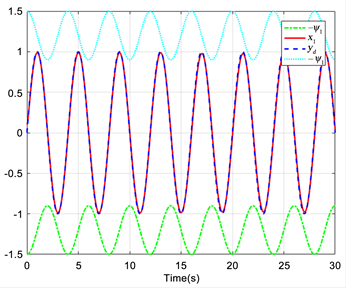Figure 1. The trajectories of system tracking performance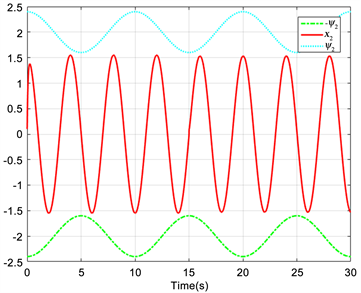Figure 2. The trajectories of system state x2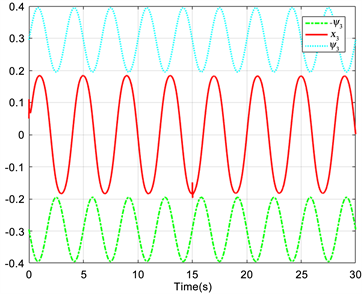Figure 3. The trajectories of system state x3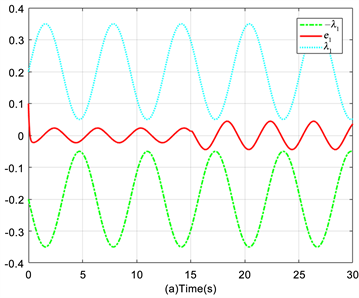Figure 4. The trajectories of tracking error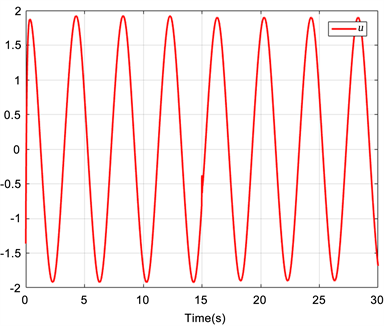Figure 5. The trajectories of control input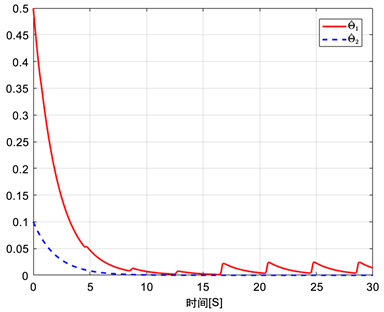Figure 6. The trajectories of adaptive laws

5. 结论